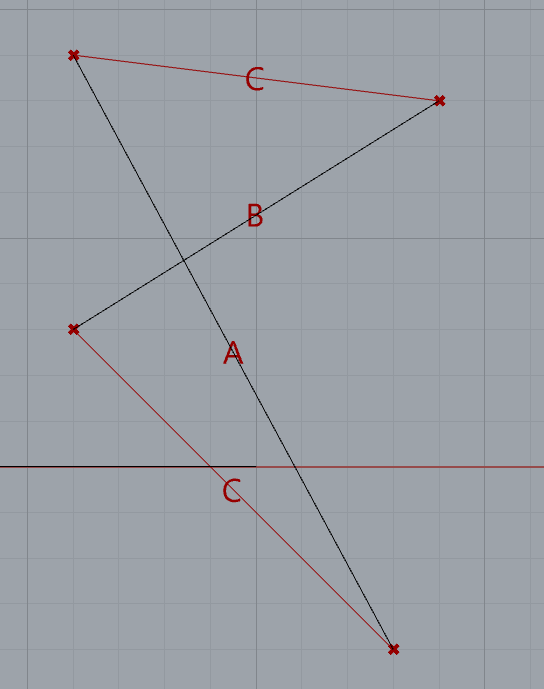# Geometry-related problem with Triangle Trigonometry component

Hey there,
I have created a definition, to return the intersection angle between any 2 given lines. The angle, in case the lines have one intersection point, has to be two by two equal. But the component returns two different values for such angles. Could you help me demystify this simple, yet confusing issue?The problem arises because the sides input to the Triangle Trig component need to be the sides of a triangle and A and B are longer than that.

Split the lines at their intersection to get the true triangles and the angles output will agree:

Triangles.gh (19.6 KB)
(I stuck the point components in only so that you can highlight each to see which it is. they are not needed for the solution but help to understand the model.)

Regards
Jeremy

1 LikeThank you @jeremy5

1 Like